# QUADRATIC EQUATION - NATURE OF ROOTS - ROOTS UNDER PARTICULAR CASES

QUADRATIC EQUATION - NATURE OF ROOTS - ROOTS UNDER PARTICULAR CASES

Let the quadratic equation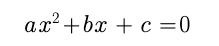has real roots and

a. if b = 0    then roots are equal in magnitude but opposite in sign

b. if c = 0.   then one root is zero other is – b/a

c. if a = c    then roots are reciprocal to each other

d. If a+b+c=0  i.e one root is 1 and second root is c/a  or(–b–a)/a.

e. if sign of a = sign of b != sign of c  then Greater root in magnitude is negative

f. if sign of b = sign of c != sign of a then Greater root in magnitude is positive

Example :

If equation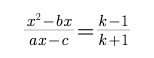has  roots equal in magnitude & opposite in sign, then the value of k is?

Solution :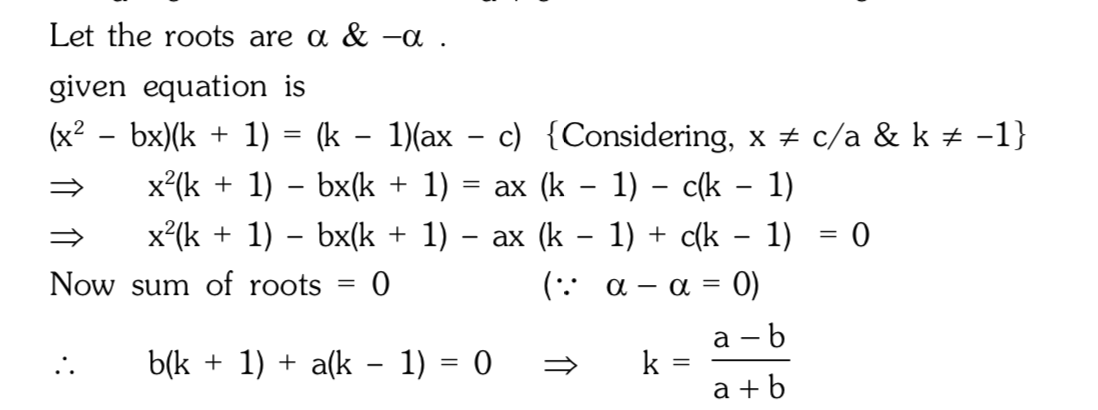Example

If roots of the equation (a–b)x2 +(c–a)x+(b–c)=0 are equal,then a,b,c are in.

Solution

Sum of the coefficients = 0

Hence one root is 1 and other root is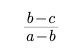Given that both roots are equal, so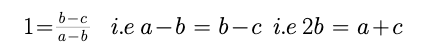Hence a, b, c are in A.P.

Post By : Rahul Kumar 07 Apr, 2020 2172 views Maths